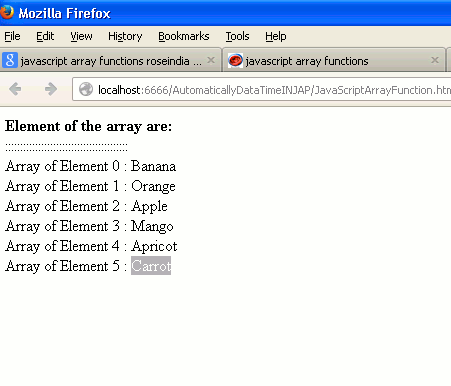Tutorials

# JavaScript array functions

We are going to describe How to create JavaScript Array function. Now we have created JavaScript Array function by help of HTML and JavaScript language.In this simple example we have used basic functionalities of JavaScript Array.

We are going to describe How to create JavaScript Array function. Now we have created JavaScript Array function by help of HTML and JavaScript language.In this simple example we have used basic functionalities of JavaScript Array.

## JavaScript array functions

We are going to describe How to create JavaScript Array function. Now we have created JavaScript Array function by help of HTML and JavaScript language.In this simple example we have used basic functionalities of JavaScript Array. This Example shows that on loading a page invoke a function display ( ).The function display includes an array variable that is used to instantiate an array. We have declare array of elements. The array object hold the different element defined by array index from  -.The for loop execute and run the script till the length i is less than the length of an array. The document. write print the element of the array that it hold. And After that display of Array of Element on Web Browser.

Example of JavaScript array Function

```<!DOCTYPE HTML PUBLIC "-//W3C//DTD HTML 4.01 Transitional//EN">
<html>
<title>javaScript Array function</title>
<meta http-equiv="Content-Type" content="text/html; charset=UTF-8"/>
<script>
function display(){
var array = new Array();
array = "Banana";
array = "Orange";
array = "Apple";
array = "Mango";
array = "Apricot"
array = "Carrot"
document.write("<b>Element of the array are:</b><br>");
document.write(":::::::::::::::::::::::::::::::::::::::::<br>");

for(i=0;i<array.length;i++){
document.write(" Array of Element "+i+" : "+array[i]+"<br>");
}
}
</script>